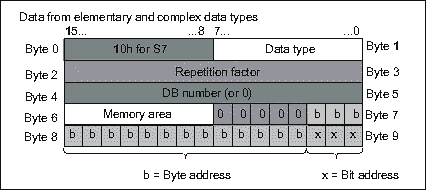# Step 7: ANY pointer parameter

When writing a function / function block, you need to determine what parameter types you will expect. Sometimes, it would be nice to be able to determine that depending on the application.

For example, in the post Step 7: analog signal normalization the output parameter could be a pseudo percentage of type INT or a motor current of type REAL.

You could write multiple functions and change the interface to match the parameter types needed, but you can hardly call that efficient. Like anything, it is easy once you know how to do that.

If you look at network 2, we already have the resulting output value as a REAL. We make a determination whether we leave that or convert it to INT or DINT. Before you start, you need to know the format of the 10-byte ANY (elementary) data type:Screenshot from the Step 7 F1 help.

Byte 0
Always 0x10 for Step 7.

Byte 1
Data type. Here, we use INT (b#16#5), DINT (b#16#7), and REAL (b#16#8).

Byte 2+3
Repetition factor (here, always 1).

Byte 4+5
DB number, or 0 for non-DB addresses like global memory (M).

Byte 6
Memory area.

Byte 7+8+9
Value.

First, we point AR1 to the address of the parameter.

```      L     P##Scaled_Out
LAR1
```

Then we analyze byte 1, indicating the data type. In our case, we test for INT, DINT, or REAL. If none of these, we skip to the end and the output value is invalid.

```      L     B [AR1,P#1.0]               // Check if destination is INT
L     5
==I
=     #OutType_int

L     B [AR1,P#1.0]               // Check if destination is DINT
L     7
==I
=     #OutType_dint

L     B [AR1,P#1.0]               // Check if destination is REAL
L     8
==I
=     #OutType_real
```

The word comprised of bytes 4+5 contains the DB number. If we are using a global memory address (MW or MD) this will be 0.

```      L     W [AR1,P#4.0]               // Obtain DB nbr
T     #Out_DB
```

We open the referenced DB. If this value is 0, Step 7 will interpret that to be a non-DB address (like M, I, Q, or L etc). Then we obtain the bytes 6+7+8+9 and point AR1 to that address.

```      OPN   DB [#Out_DB]                // Open DB
L     D [AR1,P#6.0]               // Obtain 4-byte pointer to value
```

Depending on the type of address we detected we jump to the appropriate part of the network. If the adress type is unsupported we skip tot the end. You could reflect the error in the ENO bit, for example.

```      A     #OutType_int                // If type is INT, go there
JC    ti

A     #OutType_dint               // If type is DINT, go there
JC    td

A     #OutType_real               // If type is REAL, go there
JC    tr

JU    tx                          // Skip if neither
```

Convert the REAL value that we wish to send to the output to the appropriate format, and skip to the end.

```// --- Type = int ---
ti:   L     #Out_real                   // Read output value
RND                               // Convert output REAL to INT
T     W [AR1,P#0.0]               // Write to destination

JU    tx                          // Done

// --- Type = dint ---
td:   L     #Out_real                   // Read output value
RND
T     D [AR1,P#0.0]               // Write to destination

JU    tx                          // Done

// --- Type = real ---
tr:   L     #Out_real                   // Read output value
T     D [AR1,P#0.0]               // Write to destination

tx:   NOP   0
```

And that's how easy this is. I use this all the time, very handy.

## Caveat when using global memory (M)

One very important thing to consider is this. When using a global address (M) for an input or output, the variable type must be properly assigned in the symbol table. Failure to do so will fail the type detection of the ANY pointer in the code and may result in unpredictable or unexpected results. So when intending to use a REAL output, the MD address must be defined in the symbol table as REAL.